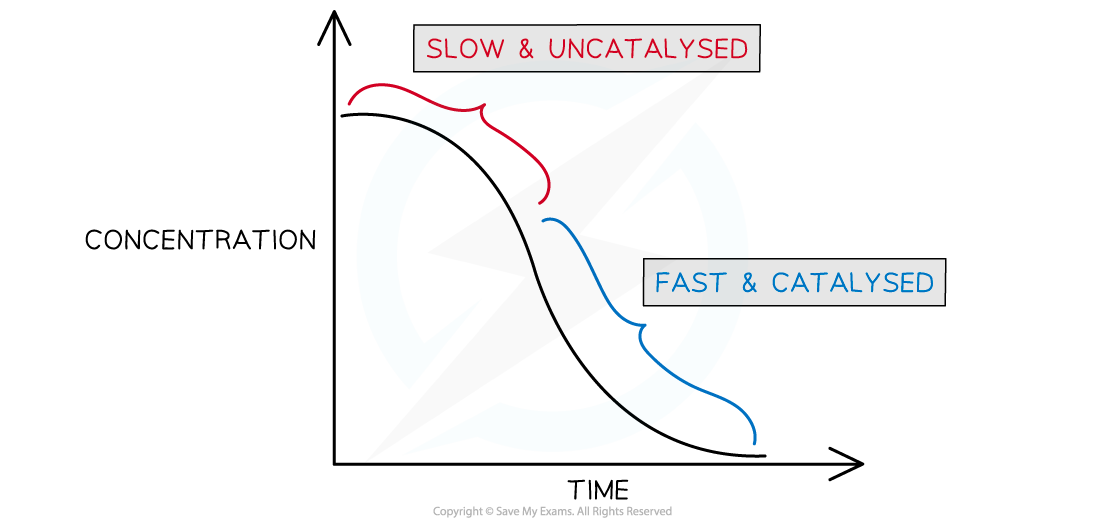# Edexcel A Level Chemistry:复习笔记6.2.12 Autocatalysis

### Manganese(II) ions as an Autocatalyst

• Autocatalysis the term used to describe a reaction which is speeded up by a product which acts as a catalyst for the reaction
• If you plot a rate graph of concentration versus time it has an usual shapeConcentration versus time for an autocatalytic reaction

• The gradient becomes steeper during the course of the reaction which tells you the rate is speeding up, not slowing down over time as the reactants become used up
• An example of an autocatalysed reaction takes place between manganate(VII) ions and oxalate (ethandioate) ions
• The overall equation can be deduced from the half equations• You can see that one of the products is manganese(II) ions - this is the catalyst
• As more manganese(II) is formed the reaction speeds up
• Like to the role of iron(II) in the previous section, manganese(II) ions take part in a redox cycle between two different oxidation states (+2 →  +3 → +2)

4Mn2+ (aq)  +  MnO4– (aq) + 8H+ (aq)   →   5Mn3+ (aq)  + 4H2O (aq)

2Mn3+ (aq)  +  C2O42- (aq)  →  2CO2 (g) +  2Mn2+ (aq)

• The manganese(II) is not present in the beginning of the reaction, but as it is formed is speeds up the reaction and is re-generated during the redox cycle
• This reaction is easily followed on a colorimeter as the rate at which the purple manganate(VII) ion is consumed accelerates with time